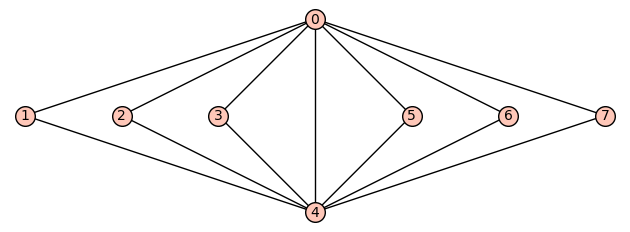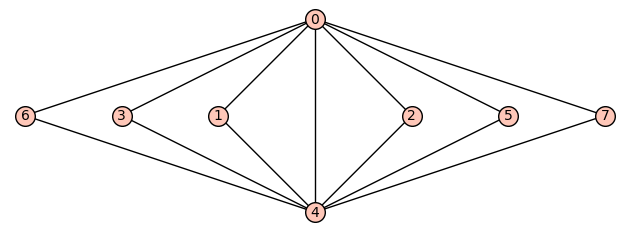# How to draw the following graph in sagemath?

How to draw the following graph in sagemath?

https://imgur.com/Q3sUXn8

I know how to draw a graph but I am finding it difficult to draw the graph in exactly the same way as shown in the picture.

Is it possible to do this?

I will be very grateful if someone could kindly help me out

edit retag close merge delete

It looks like you've asked the same question on LaTeX Stack Exchange here. LaTeX, through the tkz-berge package, is probably the best way to specify the position to create a diagram.

Sort by » oldest newest most voted

If G is you graph, you can define coordinates of the vertices by using the set_pos method, see:

sage: G.set_pos?


for more details.

EDIT Having a look at the set_pos documentation, it is actually lacking meaningful examples, so here is how to use set_pos: you have to define a dictionary that associates to each vertex a coordinate (i.e. a tuple of two numbers), as follows:

sage: G = Graph(3)
sage: G.set_pos({0:(0,0), 1:(1,1), 2:(0,1)})
sage: G.plot()

more

A better answer can be obtained via a clever use of $\LaTeX$ and Graphviz. See the relevant documentation.

1

I consider Python/Sagemath a better programming language than LaTeX, so it is easier to produce a graph with Sage and then convert it into latex/tikz, with:

sage: latex(G)


This is especially true for complex graphs.

Thierry, that's exactly what I thought of ; but my expresson wasn't exactly precise...

Alternate solution:

sage: G=Graph([[0,u] for u in (1..7)])
sage: G.add_edges([[u,4] for u in (1..3)])
sage: G.add_edges([[u,4] for u in (5..7)])
sage: D={0:[4,2]}
sage: D.update({4:[4,0]})
sage: for u in (1..3): D.update({u:[u,1]})
sage: for u in (5..7): D.update({u:[u,1]})
sage: G.plot(pos=D)EDIT : If you insist on the order of the nodes:

sage: Dpos=[4, 3, 5, 2, 4, 6, 1, 7]
sage: G.plot(pos={u:[Dpos[u], 1+(u==0)-(u==4)] for u in (0..7)})more

You define the same pos dictionary to provide coordinates to the vertices, how is it alternate ?